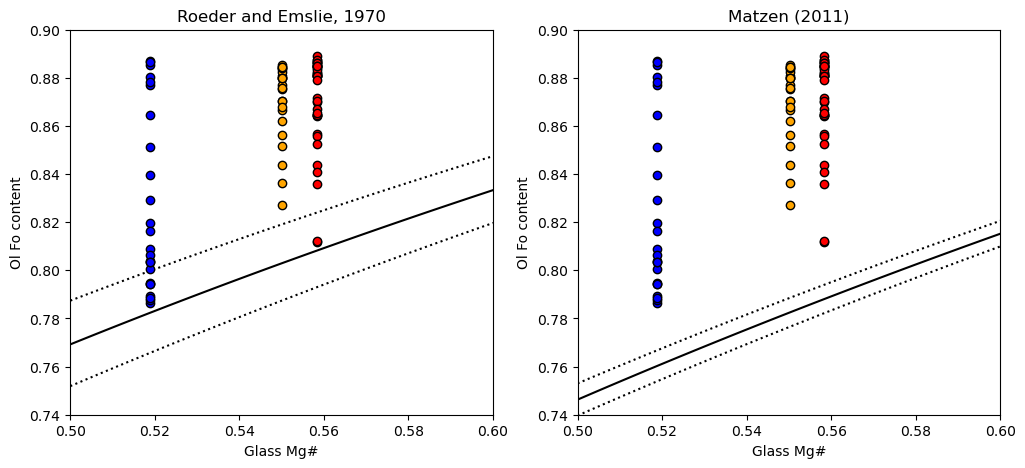This page was generated from docs/Examples/Liquid_Ol_Liq_Themometry/Olivine_MatrixGlass_Mg_Fe_Eq_MultipleSamples.ipynb. Interactive online version:.

# Multisample - Fe-Mg equilibrium between olivines and glass.

## Install Thermobar if you haven’t already (remove # and press run)

:

# !pip install Thermobar

:

# Loading various python things
import numpy as np
import pandas as pd
import matplotlib.pyplot as plt
import Thermobar as pt


### Load in the matrix glasses

:

# We dont have to add _Liq as its all liquids,
# just specify the _Liq in the import
MG_input=pt.import_excel('2018_Olivines_Glasses.xlsx',
sheet_name='Matrix_Glasses', suffix="_Liq")
MG_all=MG_input['my_input'] ## All columns
MG_Liqs=MG_input['Liqs'] ## Just Liquid columns

:

SiO2_Liq TiO2_Liq Al2O3_Liq FeOt_Liq MnO_Liq MgO_Liq CaO_Liq Na2O_Liq K2O_Liq Cr2O3_Liq P2O5_Liq H2O_Liq Fe3Fet_Liq NiO_Liq CoO_Liq CO2_Liq Sample_ID_Liq
0 50.6243 2.8773 13.0360 11.8288 0.1830 5.8471 10.2471 2.5120 0.5234 0.0 0.2689 0.0 0.0 0.0 0.0 0.0 0
1 50.0975 2.8840 12.8885 11.2897 0.1769 5.8521 9.9658 2.4681 0.5305 0.0 0.2652 0.0 0.0 0.0 0.0 0.0 1
2 50.1517 2.5827 13.0277 11.2420 0.2141 6.6763 10.6820 2.2884 0.4462 0.0 0.2068 0.0 0.0 0.0 0.0 0.0 2
3 50.2523 2.5686 13.1034 10.9155 0.1948 6.4934 10.7276 2.3660 0.4822 0.0 0.2329 0.0 0.0 0.0 0.0 0.0 3
4 51.1145 2.6104 13.2432 11.1522 0.1738 6.5764 10.6298 2.4155 0.4704 0.0 0.2393 0.0 0.0 0.0 0.0 0.0 4

### Load in the olivine data

:

Ols_in=pt.import_excel('2018_Olivines_Glasses.xlsx',
sheet_name='MIs_Ols')
Ols_Ol=Ols_in['Ols']
Ols_all=Ols_in['my_input']


### Find each sample for matrix glasses Glasses

:

MGs_LL4_index=MG_all['SpecificID']=="LL4"
MGs_LL7_index=MG_all['SpecificID']=="LL7"
MGs_LL8_index=MG_all['SpecificID']=="LL8"


### Find each sample for olivines and their melt inclusions

:

Ols_LL4_index=Ols_all['SpecificID']=="LL4"
Ols_LL7_index=Ols_all['SpecificID']=="LL7"
Ols_LL8_index=Ols_all['SpecificID']=="LL8"


## 1. Calculate Mg# for liquids

:

Liq_Mgno_calc=pt.calculate_liq_mgno(liq_comps=MG_Liqs, Fe3Fet_Liq=0.15)

# Calculate mean value to plot for each sample
Liq_Mgno_calc_mean_LL4=np.mean(Liq_Mgno_calc.loc[MGs_LL4_index])
Liq_Mgno_calc_mean_LL7=np.mean(Liq_Mgno_calc.loc[MGs_LL7_index])
Liq_Mgno_calc_mean_LL8=np.mean(Liq_Mgno_calc.loc[MGs_LL8_index])


## 2. Calculate Olivine Fo contents

:

Ol_Fo_Calc=pt.calculate_ol_fo(ol_comps=Ols_Ol)


## 3. Calculate fields you want to plot on a Rhodes diagram

:

Rhodes=pt.calculate_ol_rhodes_diagram_lines(Min_Mgno=0.5, Max_Mgno=0.7)

:

Mg#_Liq Eq_Ol_Fo_Roeder (Kd=0.3) Eq_Ol_Fo_Roeder (Kd=0.27) Eq_Ol_Fo_Roeder (Kd=0.33) Eq_Ol_Fo_Matzen (Kd=0.34) Eq_Ol_Fo_Matzen (Kd=0.328) Eq_Ol_Fo_Matzen (Kd=0.352)
0 0.500000 0.769231 0.787402 0.751880 0.746269 0.753012 0.739645
1 0.502020 0.770662 0.788751 0.753384 0.747796 0.754512 0.741198
2 0.504040 0.772087 0.790095 0.754883 0.749317 0.756006 0.742745
3 0.506061 0.773506 0.791432 0.756375 0.750832 0.757493 0.744286
4 0.508081 0.774919 0.792763 0.757861 0.752341 0.758975 0.745822

## 4. Plot the Kd model you want, along with the olivine and glass Mg

:

fig, (ax1, ax2) = plt.subplots(1, 2, figsize=(12,5))

# Plotting for Roeder and Emslie
ax1.set_title('Roeder and Emslie, 1970')
# Plotting equilibrium lines
ax1.plot(Rhodes['Mg#_Liq'], Rhodes['Eq_Ol_Fo_Roeder (Kd=0.27)'], ':k')
ax1.plot(Rhodes['Mg#_Liq'], Rhodes['Eq_Ol_Fo_Roeder (Kd=0.33)'], ':k')
ax1.plot(Rhodes['Mg#_Liq'], Rhodes['Eq_Ol_Fo_Roeder (Kd=0.3)'], '-k')

# Plotting data

ax1.plot(Ol_Fo_Calc.loc[Ols_LL4_index]*0+Liq_Mgno_calc_mean_LL4,
Ol_Fo_Calc.loc[Ols_LL4_index], 'ok', mfc='blue')

ax1.plot(Ol_Fo_Calc.loc[Ols_LL7_index]*0+Liq_Mgno_calc_mean_LL7,
Ol_Fo_Calc.loc[Ols_LL7_index], 'ok', mfc='orange')

ax1.plot(Ol_Fo_Calc.loc[Ols_LL8_index]*0+Liq_Mgno_calc_mean_LL8,
Ol_Fo_Calc.loc[Ols_LL8_index], 'ok', mfc='red')

ax2.set_title('Matzen (2011)')
# Plotting equilibrium lines
ax2.plot(Rhodes['Mg#_Liq'], Rhodes['Eq_Ol_Fo_Matzen (Kd=0.328)'], ':k')
ax2.plot(Rhodes['Mg#_Liq'], Rhodes['Eq_Ol_Fo_Matzen (Kd=0.352)'], ':k')
ax2.plot(Rhodes['Mg#_Liq'], Rhodes['Eq_Ol_Fo_Matzen (Kd=0.34)'], '-k')
# Plotting data
ax2.plot(Ol_Fo_Calc.loc[Ols_LL4_index]*0+Liq_Mgno_calc_mean_LL4,
Ol_Fo_Calc.loc[Ols_LL4_index], 'ok', mfc='blue')

ax2.plot(Ol_Fo_Calc.loc[Ols_LL7_index]*0+Liq_Mgno_calc_mean_LL7,
Ol_Fo_Calc.loc[Ols_LL7_index], 'ok', mfc='orange')

ax2.plot(Ol_Fo_Calc.loc[Ols_LL8_index]*0+Liq_Mgno_calc_mean_LL8,
Ol_Fo_Calc.loc[Ols_LL8_index], 'ok', mfc='red')
ax1.set_ylabel('Ol Fo content')
ax2.set_ylabel('Ol Fo content')
ax1.set_xlabel('Glass Mg#')
ax2.set_xlabel('Glass Mg#')


:

(0.5, 0.6)